Levels »

# Warning: Earthquake Zone

### The Pit, Elevation: -22.7m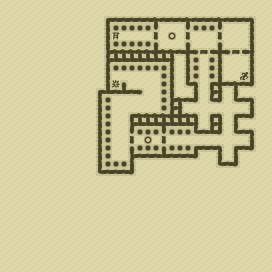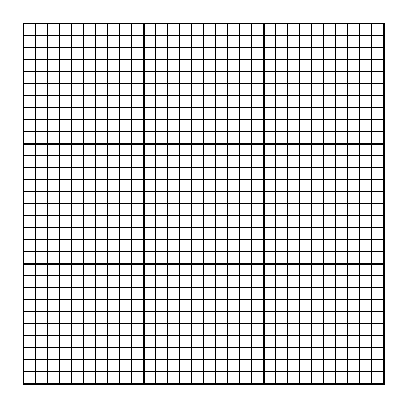### Items

•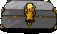Lead Box (13, 11)
•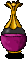Pale Violet Potion (18, 8)
•Ruby Ring (13, 11) (in Lead Box)
•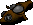Gas Mask (29, 8) (on Juan)
•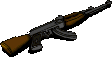3 AK-47 Assault Rifles (29, 8) (on Juan) - (24, 12) - (27, 14)
•6 AK-47 Magazines (134 rounds) (29, 8) x19 (in AK-47 Assault Rifle) (on Juan) - (29, 8) x30 (on Juan) - (29, 8) x30 (on Juan) - (29, 8) x30 (on Juan) - (24, 12) x19 (in AK-47 Assault Rifle) - (27, 14) x6 (in AK-47 Assault Rifle)
•AK-47 SABOT Magazine (30 rounds) (27, 8) x30

•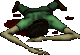(29, 8) Juan
•AK-47 Assault Rifle
•AK-47 Magazine x19
•AK-47 Magazine x30
•AK-47 Magazine x30
•AK-47 Magazine x30
•Gas Mask

### Monsters

•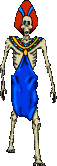45 Ghasts (Random rate: 5) (19, 2) - (21, 2) - (27, 2) - (15, 3) - (24, 3) - (28, 3) - (19, 4) - (21, 4) - (23, 4) - (25, 4) - (27, 4) - (27, 6) - (29, 6) - (24, 7) - (27, 7) - (13, 8) - (15, 8) - (15, 8) - (16, 8) - (24, 8) - (15, 9) - (17, 9) - (18, 9) - (17, 10) - (18, 10) - (14, 11) - (15, 11) - (18, 11) - (13, 12) - (16, 12) - (17, 12) - (13, 13) - (14, 13) - (14, 14) - (13, 15) - (13, 16) - (14, 16) - (16, 16) - (17, 16) - (18, 16) - (20, 16) - (21, 16) - (21, 16) - (22, 16) - (14, 17)
•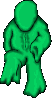6 Green Oozes (27, 10) - (22, 12) - (29, 12) - (24, 14) - (29, 16) - (27, 18)
•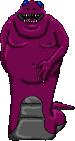Malice (15, 12)

### Locations

• 5 Door closers (19, 3) - (23, 3) - (24, 6) - (14, 16) - (18, 16)
• 6 Door openers (17, 3) - (21, 3) - (25, 3) - (24, 4) - (28, 4) - (16, 16)
• 20 Do-not-disturb sectors(13, 8) - (14, 8) - (15, 8) - (16, 8) - (17, 8) - (18, 8) - (15, 9) - (16, 9) - (17, 9) - (18, 9) - (17, 10) - (18, 10) - (15, 11) - (16, 11) - (17, 11) - (18, 11) - (15, 12) - (16, 12) - (17, 12) - (18, 12)
• 2 Save runes(20, 3) - (17, 16)